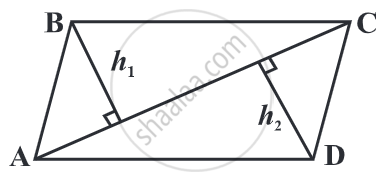# Area of a General Quadrilateral

#### formula

Area of quadrilateral = 1/2 d (height1 + height2) where d denotes the length of diagonal.

# Area of a General Quadrilateral:

A general quadrilateral can be split into two triangles by drawing one of its diagonals. This "triangulation" helps us to find a formula for any general quadrilateral.Area of quadrilateral ABCD = (area of ∆ABC) + (area of ∆ADC)

= (1/2 xx "AC" xx h_1) + (1/2 xx "AC" xx h_2)

= 1/2 × AC × (h1 + h2)

= 1/2 d (h1 + h2) where d denotes the length of diagonal AC.

If you would like to contribute notes or other learning material, please submit them using the button below.

### Shaalaa.com

Area of a General Quadrilateral [00:06:33]
S
0%